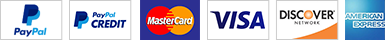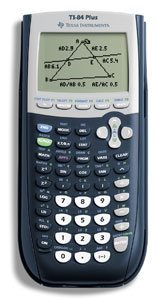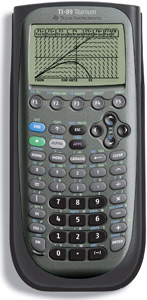Math ResourcesHome  Algebra  Geometry  Arithmetic  Trigonometry  Probability/Statistics  Calculus  Mechanics GED  SAT  ACT  College Mathematics  GRE  School Supplies  About Usand Practice

The Math Tutor welcomes you. The objective here is to get you passing your Math exam or even if you only just want to brush up on
your math. The strategy is simply through practicing. You have to put pen and paper together and practice how to solve problems.
Tutoring without practicing on your own will not help. This is what will happen starting from the beginning of the Course Content and for each topic
as we move along:

1. An introduction or preamble will be given with worked examples.
2. A set of exam-style questions will follow for you to solve.
3. You will try to solve all problems as instructed while showing what you have done.
(That's where the practice will come in.)
4.
Scan or take a photo of your work.
5. Send work in by email with photo attached.
6.
Tutor corrects your work by pointing out mistakes and showing how to solve each problem.
7. Work sent back to you for review and keep as reference.
8. We move on to the next topic.

There will be no need to worry about getting anything right or wrong because our job is to find mistakes and correct them so that you can be able
to solve more problems, including those of your exam.
Our math workout should take about 6 weeks or more.

The email address you provide will be our means of communication. The Tutor will also provide at no extra cost software applications that will be

Choose a Math Workout below from GED, SAT 1, SAT 2, and ACT.                                                                                                                                                            A Proven Success

Email:

 GED Math Workout - (US)\$50.00ACT Math Workout - (US)\$50.00SAT Math 1 Workout - (US)\$50.00SAT Math 2 Workout - (US)\$50.00Applications  for High School Students Applications will calculate for you while giving an understanding of each topic involved. Downloads only for Windows Instructions will be sent to you through the email provided within 24 hrs. of purchase.
 Linear Equations - (US)\$4.99 Equations and GraphsExponential and Logarithmic Calculations - (US)\$4.99  Common Logarithms, Decay Factor, Exponential Function Exponential and Logarithmic Equations, Growth Factor Fractional Exponents, Laws of Exponents, Negative Exponents Natural Logarithms, Principles of Exponents, Properties of LogarithmsPermutations and Combinations - (US)\$4.99 Factorials, Permutations with Like and Unlike Objects, Combinations Permutations with and Without Replacement, Circular PermutationsQuadratic Solutions - (US)\$4.99 Equations and GraphsSimple and Compound Interests - (US)\$4.99 Principal, Rate, Time, Present Value, Future ValueBasic Financial Math Calculations - (US)\$9.99 Amortization, Annual Percentage Rate, Annuities, Arithmetic Mean, Arithmetic Progression Compound Interest, Simple Interest, Effective Annual Yield,  Exponents, Future Value Geometric Progression, Geometric Mean, Infinite Geometric Series, Present Value Percent, Ratio and Proportions, Sinking Funds, Sum to Infinity . . . more.Algebra Calculations - (US)\$14.99 Algebra calculations in one place. Radians, Arcs and Sectors, Reciprocals, Percent, Factorials Permutations, Combinations, Simple and Compound Interests Order of Operations, Ratio and Proportions Co-ordinates and the Straight Line, Length of a Straight Line Mid-point of a Straight Line, Gradient of a Straight Line, Equation of a Straight Line Graphs of a Straight Line, Logarithms, Pythagorean Theorem Geometric Progression, Arithmetic Progression, Harmonic Progression Arithmetic Sequence,  Arithmetic Mean, Common Difference, Sum of Arithmetic Series Geometric Sequence, Common Ratio, Sum of Geometric Series Quadratic Solutions, Exponents (Indices), Negative Exponents Fractional Exponents, Laws of Exponents, Natural Logarithms, Exponential Function Common Logarithms, Antilogarithms, Summation Notation Inequalities, Simple EquationsGeometry Calculations - (US)\$14.99 Geometry calculations in one place. Congruent Triangles, Similar Triangles, Area of Triangle Perimeter of Triangle, RATIO and PROPORTIONS, Equation of a Circle Parallel Lines, Perpendicular Lines, Pythagorean Theorem RADIANS, ARCS and SECTORS, Length of an Arc, Area of a Sector PLANE FIGURES, SOLID FIGURES, The Square, Rectangle Triangles, Circle, Trapezoid, Parallelogram, Prisms Square Prism, Rectangular Prism, Right Circular Cylinder Sphere, Pyramids, Square Pyramid, Triangular Pyramid, Cone POINTS, LINES and ANGLES, Hypotenuse, CO-ORDINATES THE STRAIGHT LINE, Complementary Angles, Supplementary Angles Adjacent Angles, Vertically Opposite Angles, Alternate Angles Corresponding Angles, Mid-point of a straight line Slope of a straight line, Length of a straight line Graphs of a straight line, Equation of a straight line, Polygonsfor Math Practice, GED  SAT  ACT  College Math  GRE

 Cheap Calculators and ComputersMore School Supplies and other useful items *  * *   *